# Test: Word Problems - 1

## 15 Questions MCQ Test Practice Questions for GMAT | Test: Word Problems - 1

Description
Attempt Test: Word Problems - 1 | 15 questions in 30 minutes | Mock test for GMAT preparation | Free important questions MCQ to study Practice Questions for GMAT for GMAT Exam | Download free PDF with solutions
QUESTION: 1

### A political candidate collected \$1,749 from a fund raising dinner. If each supporter contributed at least \$50, what is the greatest possible number of contributors at the dinner?

Solution:

To determine the greatest possible number of contributors we must assume that each of these individuals contributed the minimum amount, or \$50.  We can then set up an inequality in which n equals the number of contributors:
50n is less than or equal to \$1,749 Divide both sides of the equation by 50 to isolate n, and get n is less than or equal to 34.98
Since n represents individual people, it must be the greatest whole number less than 34.98.  Thus, the greatest possible value of n is 34.
Alternately, we could have assumed that the fundraiser collected \$1,750 rather than \$1,749.  If it had, and we assumed each individual contributed the minimum amount, there would have been exactly 35 contributors (\$50 x 35 = \$1,750).  Since the fundraiser actually raised one dollar less than \$1,750, there must have been one fewer contributor, or 34.

QUESTION: 2

### Joan, Kylie, Lillian, and Miriam all celebrate their birthdays today. Joan is 2 years younger than Kylie, Kylie is 3 years older than Lillian, and Miriam is one year older than Joan. Which of the following could be the combined age of all four women today?

Solution:

It may be easiest to represent the ages of Joan, Kylie, Lillian and Miriam ( J, K, L and M) on a number line. If we do so, we will see that the ages represent consecutive integers as shown in the diagram.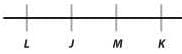Since the ages are consecut ive integers, they can all be expressed in terms of L: L, L + 1, L + 2, L + 3. The sum of the four ages then would be 4L + 6. Since L must be an integer (it’s Lillian’s age), the expression 4L + 6 describes a number that is two more than a multiple of 4:
4L + 6 = (4L + 4) + 2
[4L + 4 describes a mult iple of 4, since it can be factored into 4( L + 1) or 4 * an integer.]
54 is the only number in the answer choices that is two more than a multiple of 4 (namely, 52).

QUESTION: 3

### Janet is now 25 years younger than her mother Carol. If in 6 years Janet's age will be half Carol’s age, how old was Janet 5 years ago?

Solution:

This is an algebraic translat ion problem dealing with ages. For this t ype of problem, an age chart can help us keep track of the variables: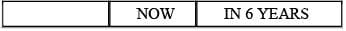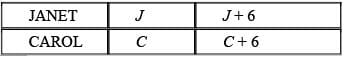Using the chart in combination with the statements given in the question, we can derive equations to relate the variables. The first statement tells us that Janet is now 25 years younger than her mother Carol. Since we have used J to represent Janet’s current age, and C to represent Carol’s current age, we can translate the statement as follows: J = C – 25.
The second statement tells us that Janet will be half Carol’s age in 6 years. Since we have used (J + 6) to represent Janet’s age in 6 years, and (C + 6) to represent Carol’s age in 6 years, we can translate the statement as follows: J + 6 = (1/2)(C + 6).
Now, we can subst itute the expression for C (C = J + 25) derived from the first equation into the second equation (note: we choose to subst itute for C and solve for J because the question asks us for Janet's age 5 years ago):
J + 6 = (1/2)(J + 25 + 6)
J + 6 = (1/2)(J + 31)
2J + 12 = J + 31
J = 19
If Janet is now 19 years old, she was 14 years old 5 years ago.

QUESTION: 4

A certain company has budgeted \$1,440 for entertainment expenses for the year, divided into 12 equal monthly allocations. If by the end of the third month, the total amount spent on entertainment was \$300, how much was the company under budget or over budget?

Solution:

The \$1,440 is divided into 12 equal monthly allocations.
1440/12 = \$120
The company has \$120 allocated per month for entertainment, so the allocation for three months is 120 × 3 = 360
Since the company has spend a total of \$300 t hus far, it is \$360 - \$300 = \$60 under budget.

QUESTION: 5

The ACME company manufactured x brooms per month from January to April, inclusive. On the first of each month, during the following May to December, inclusive, it sold x/2 brooms. At the beginning of production on January 1st, the ACME company had no brooms in its inventory. If storage costs were \$1 per month per broom, approximately how much, in terms of x, did the ACME company pay for storage from May 2nd to December 31st, inclusive?

Solution:

Since this problem includes variables in both the question and the answer choices, we can try solving by plugging in smart numbers. For x, we want to choose a multiple of 2 because we will have to take x/2 later. Let's say that ACME produces 4 brooms per month from January to April, so x = 4. The total number of brooms produced was (4 brooms x 4 months), or 16 brooms. ACME sold x/2 brooms per month, or 2 brooms per month (because we chose x = 4). Now we need to start figuring out the storage costs from May 2 nd to December 31st. Since ACME sold 2 brooms on May 1st, it needed to store 14 brooms that month, at a cost of \$14. Following the same logic, we see that ACME sold another t wo brooms June 1st and stored 12 brooms, which cost the company \$12. We now see that the July storage costs were \$10, August were \$8, September \$6, October \$4, November \$2, and for December there were no storage costs since the last 2 brooms were sold on December 1st. So ACME's total storage costs were 14 + 12 + 10 + 8 + 6 + 4 + 2 = \$56. Now we just need to find the answer choice that gives us \$56 when we plug in the same value, x = 4, that we used in the question. Since 14 x 4 = 56, \$14x must be the correct value. The correct answer is E.
While plugging in smart numbers is the preferred method for VIC problems such as this one, it is not the only method. Below is an alternative, algebraic method for solving this problem: ACME accumulated an inventory of 4x brooms during its four-month production period. If it sold 0.5x brooms on May 1st , then it paid storage for 3.5x brooms in May, or \$3.5x. Again, if ACME sold 0.5x brooms on June 1st, it paid storage for 3x brooms in June, or \$3x. The first row of the table below shows the amount of money spent per month on storage. Notice that since ACME liquidated its stock on December 1st, it paid zero dollars for storage in December.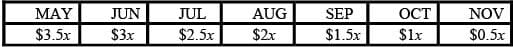f we add up these costs, we see that ACME paid \$14x for storage.

QUESTION: 6

The number of passengers on a certain bus at any given time is given by the equation P = -2(S – 4)2 + 32, where P is the number of passengers and S is the number of stops the bus has made since beginning its route. If the bus begins its route with no passengers, how many passengers will be on the bus two stops after the stop where it has its greatest number of passengers?

Solution:

The bus will carry its greatest passenger load when P is at its maximum value. If P = -2(S – 4)2 + 32, the maximum value of P is 32 because (S – 4)2 will never be negat ive, so the expression -2(S – 4)2 will never be positive. The maximum value for P will occur when -2(S – 4)2 = 0, i.e. when S = 4.
The question asks for the number of passengers two stops after the bus reaches its greatest passenger load, i.e. after 6 stops (S = 6).
P = -2(6 – 4)2 + 32
P = -2(2)2 + 32
P = -8 + 32
P = 24
Alternat ively, the maximum value for P can be found by building a table, as follows: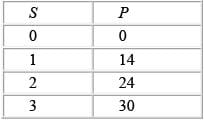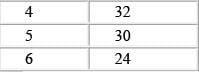The maximum value for P occurs when S = 4. Thus, two stops later at S = 6, P = 24.

QUESTION: 7

John was 27 years old when he married Betty. They just celebrated their fifth wedding anniversary, and Betty's age is now 7/8 of John's. How old is Betty?

Solution:

John was 27 when he married Betty, and since they just celebrated their fift h wedding anniversary, he is now 32.
Since Betty's age now is 7/8 of John's, her current age is (7/8) × 32, which equals 28.

QUESTION: 8

Joe needs to paint all the airplane hangars at the airport, so he buys 360 gallons of paint to do the job. During the first week, he uses 1/4 of all the paint. During the second week, he uses 1/5 of the remaining paint. How many gallons of paint has Joe used?

Solution:

Joe uses 1/4 of 360, or 90 gallons, during the first week. He has 270 gallons remaining (360 –90 = 270).
During the second week, Joe uses 1/5 of the remaining 270 gallons, which is 54 gallons.
Therefore, Joe has used 144 gallons of paint by the end of the second week (90 + 54 = 144).

QUESTION: 9

A certain movie star's salary for each film she makes consists of a fixed amount, along with a percentage of the gross revenue the film generates. In her last two roles, the star made \$32 million on a film that grossed \$100 million, and \$24 million on a film that grossed \$60 million. If the star wants to make at least \$40 million on her next film, what is the minimum amount of gross revenue the film must generate?

Solution:

One way to do this problem is to recognize that the star earned \$8M more (\$32M -\$24M = \$8M) when her film grossed \$40M more (\$100M - \$60M = \$40M). She wants to earn \$40M on her next film, or \$8M more than she earned on the more lucrative of her other two films. Thus, her next film would need to gross \$40M more than \$100M, or \$140M.
Alternatively, we can solve this problem using algebra. The star's salary consists of a fixed amount and a variable amount, which is dependent on the gross revenue of the film. We know what she earned for two films, so we can set up two equations, where f is her fixed salary and p is her portion of the gross, expressed as a decimal:
She earned \$32 million on a film that grossed \$100 million: \$32M = f + p(\$100M) She earned \$24 million on a film that grossed \$60 million: \$24M = f + p(\$60M) We can solve for p by subtracting the second equation from the first: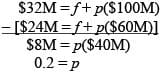We can now plug in 0.2 for p in eit her of the original equat ions to solve for f: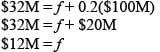Now that we know her fixed salary and the percentage of the gross earnings she
receives, we can rewrite the formula for her total earnings as: Total earnings = \$12M + 0.2(gross)
Finally, we just need to figure out how much gross revenue her next film needs to generate in order for her earnings to be \$40 million: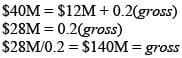QUESTION: 10

As a bicycle salesperson, Norman earns a fixed salary of \$20 per week plus \$6 per bicycle for the first six bicycles he sells, \$12 per bicycle for the next six bicycles he sells, and \$18 per bicycle for every bicycle sold after the first 12. This week, Norman earned more than twice as much as he did last week. If he sold x bicycles last week and y bicycles this week, which of the following statements must be true?
I. y > 2x
II. y > x
III. y > 3

Solution:

. UNCERTAIN: It depends on how many bicycles Norman sold.
For example, if x = 4, then Norman earned \$44 [= \$20 + (4 × \$6)] last week. In order to double his earnings, he would have to sell a minimum of 9 bicycles this week (y = 9), making \$92 [= \$20 + (6 × \$6) + (3 × \$12)]. In that case, y > 2x.
However, if x = 6 and y = 11, then Norman would have earned \$56 [= \$20 + (6 × \$6)] last week and \$116 [= \$20 + (6 × \$6) + (5 × \$12)] this week. In that case, \$116 > 2 × \$56, yet y < 2x.
So, it is possible for Norman to more than double his earnings without selling twice as many bicycles.
II. TRUE: In order to earn more money this week, Norman must sell more bicycles.
III. TRUE: If Norman did not sell any bicycles at all last week ( x = 0), then he would have earned the minimum fixed salary of \$20. So he must have earned at least \$40 this week. If y = 3, then Norman earned \$38 [= \$20 + (3 × \$6)] this week. If y = 4, then Norman earned \$44 [= \$20 + (4 × \$6)] this week. Therefore, Norman must have sold at least 4 bicycles this week, which can be expressed y > 3.

QUESTION: 11

A basketball team composed of 12 players scored 100 points in a particular contest. If none of the individual
players scored fewer than 7 points, what is the greatest number of points that an individual player might have
scored?

Solution:

In order to determine the greatest number of points that an individual player might have scored, assume that 11 of the 12 players scored 7 points, the minimum possible. The 11 low scorers would therefore account for 7(11) = 77 points out of 100. The number of points scored by the twelft h player in this scenario would be 100 – 77 = 23.

QUESTION: 12

Sally has a gold credit card with a certain spending limit, and a platinum card with twice the spending limit of the gold card. Currently, she has a balance on her gold card that is 1/3 of the spending limit on that card, and she has a balance on her platinum card that is 1/5 of the spending limit on that card. If Sally transfers the entire balance on her gold card to her platinum card, what portion of her limit on the platinum card will remain unspent?

Solution:

Since we are not given any actual spending limits, we can pick numbers. In problems involving fractions, it is best to pick numbers that are multiples of the denominators.
We can set the spending limit for the gold account at \$15, and for the platinum card at \$30. In this case, Sally is carrying a balance of \$5 (which is 1/3 of \$15) on her gold card, and a balance of \$6 (1/5 of \$30) on her plat inum card. If she transfers the balance from her gold card to her plat inum card, the plat inum card will have a balance of \$11. That means that \$19 out of her \$30 spending limit would remain unspent.
Alternat ively, we can so lve this algebraically by using the variable x to represent the spending limit on her platinum card: (1/5)x + (1/3)(1/2)x = (1/5)x + (1/6)x = (6/30)x + (5/30)x = (11/30)x
This leaves 19/30 of her spending limit untouched.

QUESTION: 13

Martina earns one-sixth of her annual income during the month of June and one-eighth in August. Pam earns one-third of her annual income in June and one-fourth in August. Martina's earnings for June and August equal Pam's earnings for the same period. What portion of their combined annual income do the two girls earn during the ten months NOT including June and August?

Solution:

The problem talks about Martina and Pam's incomes but never provides an actual dollar value, either in the quest ion or in the answer choices. We can, therefore, use smart numbers to solve the problem. Because the dollar value is unspecified, we pick a dollar value with which to solve the problem. To answer the question, we need to calculate dollar values for the portion of income each earns during the ten months not including June and August, and we also need to calculate dollar values for each player's annual income.
Let's start with Martina, who earns 1/6 of her income in June and 1/8 in August. The common denominator of the two fractions is 24, so we set Martina's annual income at \$24. This means that she earns \$4 (1/6 × 24) in June and \$3 (1/8 × 24) in August, for a total of \$7 for the two months. If Martina earns \$7 of \$24 in June and August, then she earns \$17 during the other ten months of the year. The problem tells us that Pam earns the same dollar amount during the two months as Martina does, so Pam also earns \$7 for June and August. The \$7 Pam earns in June and August represents 1/3 + 1/4 of her annual income. To calculate her annual income, we solve the equation: 7 = (1/3 + 1/4)x, with x represent ing Pam's annual income. This simplifies to 7 = (7/12)x or 12 = x. If Pam earns \$7 of \$12 in June and August, then she earns \$5 during the other ten months of the year. [NOTE: we cannot simply pick a number for Pam in the same way we did for Martina because we are given a relationship between Martina's income and Pam's income. It is a coincidence that Pam's income of \$12 matches the common denominator of the two fractions assigned to Pam, 1/3 and 1/4 -if we had picked \$48 for Martina's income, Pam's income would then have to be \$24, not \$12.]
Combined, the two players earn \$17 + \$5 = \$22 during the other ten months, out of a combined annual income of \$24 + \$12 = \$36. The portion of the combined income earned during the other ten months, therefore, is 22/36 which simplifies to 11/18. Note first that you can also calculate the portion of income earned during June and August and then subtract this fraction from 1. The portion of income earned during June and August, 7/18, appears as an answer choice, so be careful if you decide to solve it this way.
Note also that simply adding the four fractions given in the problem produces the number 7/8, an answer choice. 1/8 (or 1 – 7/8) is also an answer choice. These two answers are  "too good to be true" - that is, it is too easy to arrive at these numbers.

QUESTION: 14

On January 1, 2076, Lake Loser contains x liters of water. By Dec 31 of that same year, 2/7 of the x liters have evaporated. This pattern continues such that by the end of each subsequent year the lake has lost 2/7 of the water that it contained at the beginning of that year. During which year will the water in the lake be reduced to less than 1/4 of the original x liters?

Solution:

This fract ion problem contains an "unspecified" total (the x liters of water in the lake). Pick an easy "smart" number to make this problem easier. Usually, the smart number is the lowest common denominator of all the fractions in the problem. However, if you pick 28, you will quickly see that this yields some unwieldy computation.
The easiest number to work with in this problem is the number 4. Let's say there are 4 liters of water originally in the lake. The question then becomes: During which year is the lake reduced to less than 1 liter of water?
At the end of 2076, there are 4 × (5/7) or 20/7 liters of water in the lake. This is not less than 1.
At the end of 2077, there are (20/7) × (5/7) or 100/49 liters of water in the lake. This is not less than 1.
At the end of 2078, there are (100/49) × (5/7) or 500/343 liters of water in the lake. This is not less than 1.
At the end of 2079, there are (500/343) × (5/7) or 2500/2401 liters of water in the lake. This is not less than 1.
At the end of 2080, there are (2500/2401) × (5/7) or 12500/16807 liters of water in the lake. This is less than 1.
Notice that picking the number 4 is essent ial to minimizing the computation involved, since it is very easy to see when a fract ion falls below 1 (when the numerator becomes less than the denominator.) The only moderately difficult computation involved is multiplying the denominator by 7 for each new year.

QUESTION: 15

3/4 of all married couples have more than one child. 2/5 of all married couples have more than 3 children. What fraction of all married couples have 2 or 3 children?

Solution:

This fract ion problem contains an unspecified total (the number of married couples) and is most easily solved by a picking a "smart" number for that total. The smart number is the least common denominator of all the fract ions in the problem. In this case, the smart number is 20.
Let's say there are 20 married couples. 15 couples (3/4 of the total) have more than one child. 8 couples (2/5 of the total) have more than three children.
This means that 15 – 8 = 7 couples have eit her 2 or 3 children. Thus 7/20 of the married couples have either 2 or 3 children.Use Code STAYHOME200 and get INR 200 additional OFF Use Coupon Code